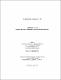## Point cover problem in 3D2017
##### Authors
Akter, Sharmin
University of Lethbridge. Faculty of Arts and Science
##### Publisher
Lethbridge, Alta. : Universtiy of Lethbridge, Department of Mathematics and Computer Science
##### Abstract
We examine the problem of covering points with minimum number of axis-parallel lines in three dimensional space which is an NP-complete problem. We introduce Lagrangian based algorithms to approximate the point cover problem. We study the Lift-and-Project relaxation of the standard IP to obtain lower bounds. This method is used to strengthen the integrality gap of a problem. Our experimental results show that the Lagrangian relaxation method gives very good lower bounds at reasonable computational cost. We present a hybrid method where the Lift-and-Project LP is solved using the Subgradient Optimisation technique. We propose an approximation algorithm which iteratively uses the Lagrangian relaxation procedure. We also study a Branch-and-Bound method which gives an optimal solution. We use a drop-in accelerator while conducting the simulations on large instances.
##### Keywords
three dimensional space , points , axis parallel lines , Lagrangian relaxation , subgradient optimisation , lift-and-project , primal-dual , iterative , branch-and-bound , NVBLAS